Math Lessons

Our "almost fun" Math lessons explain Math concepts using non-Math concepts you're already familiar with.

Use our interactive lessons and calculators to master these skills 🥳.

Numbers and Arithmetic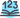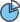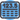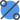Equations, Expressions, and Functions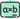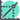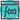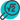Geometry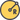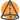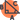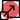Probability and Statistics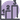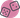Algebra I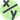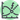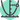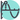Geometry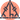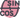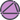Our TeamLearning ResourcesTerms & Privacy